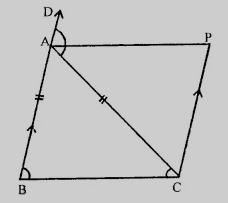"
">

# In the figure, $AB = AC$ and $CP \parallel BA$ and $AP$ is the bisector of exterior $\angle CAD$ of $\triangle ABC$. Prove that $ABCP$ is a parallelogram."

Given:

In the figure, $AB = AC$ and $CP \parallel BA$ and $AP$ is the bisector of exterior $\angle CAD$ of $\triangle ABC$.

To do:

We have to prove that $ABCP$ is a parallelogram.

Solution:

In $\triangle ABC$,

$AB =AC$

This implies,

$\angle C = \angle B$                   (Angles opposite to equal sides)

$\angle CAD = \angle B + \angle C$

$= \angle C + \angle C$

$= 2\angle C$.......….(i)

$AP$ is the bisector of $\angle CAD$

This implies,

$2\angle PAC = \angle CAD$.........…(ii)

From (i) and (ii), we get,

$\angle C = 2\angle PAC$

$\angle C = \angle CAD$

$\angle BCA = \angle PAC$

$\angle PAC$ and $\angle BCA$ are alternate angles,

This implies,

$AD \parallel BC$

$BA \parallel CP$

Therefore,

$ABCP$ is a parallelogram.

Updated on: 10-Oct-2022

25 Views